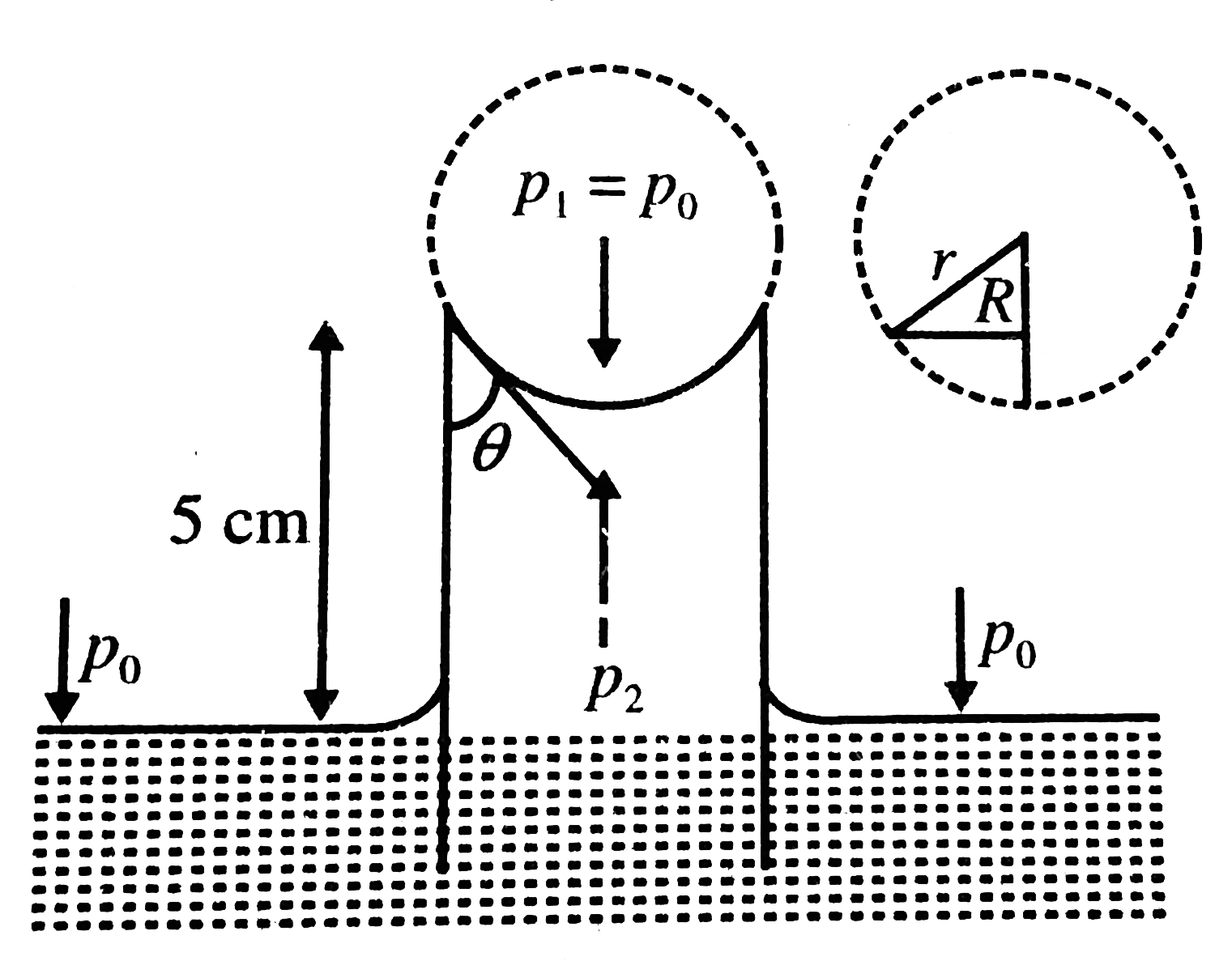# A long capillary tube of radius 0.2 mm is placed vertically inside a beaker of water. If the tube is now pushed into water so that only 5.0 cm of

69 views
in Physics
closed
A long capillary tube of radius 0.2 mm is placed vertically inside a beaker of water.
If the tube is now pushed into water so that only 5.0 cm of its length is above the surface, then determine the angle of contact between the liquid and glass surface.
A. cos^(-1)(4/5)
B. cos^(-1)(5/7)
C. cos^(-1)(3/5)
D. cos^(-1)(5/4)

by (87.1k points)
selected

Here s=7.0x10^(-2) N//m,r=0.2xx10^(-3)m,r=10^(3)kg//m^(3)
we know that the hieght of liquid in the tube is
h=2sigmacostheta//rhoRg
where R is the radius of the menisus, and theta is the angle of contact.
Since theta=0 (given), radius of the meniscus is equal to the radius of the capillary tube i.e. R=r
:. h=(2(7.0xx10^(-2)))/((10^(3))(0.2xx10^(-3))(10))=0.07m
or h=7.0cm
When the length of the capillary tube above the free surface of the liquid is less than the height of liquid that rise in the tube, radius R of the free surface is not equal to the radius of the tube. It is greater than r as the surface tennis to be flatter.
According to the equation p_(1)-p_(2)=(4sigma//R), the pressure difference across te surface is given by
/_p=(2sigmacostheta)/R
If p_(1) and p_(2) are the pressure just above and below the mensisus, respectively then p_(1)-p_(2)=rhogh_(0)
:. rhogh_(0)=(2sigma)/r...........i
In part a we have seen than when h_(0)=h,theta=0, R=r, and rhogh=(2sigma)/r.......ii
Therefore dividing eqn i by eqn ii we have r/R=(h_(0))/h
From the figure, it is clear that costheta=r/R
Therefore the angle of contact is
theta=(cos^(-1)h_(0))/h=cos^(-1)(5/7)~~44^@